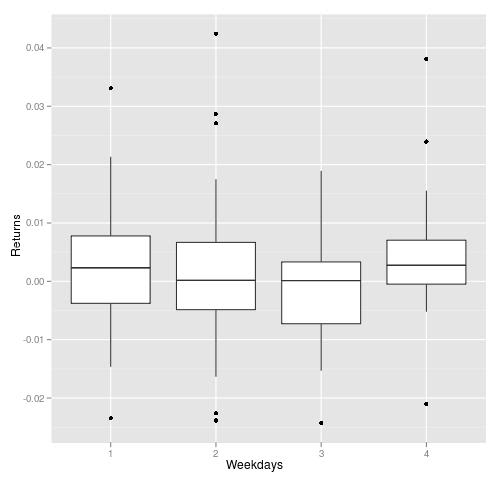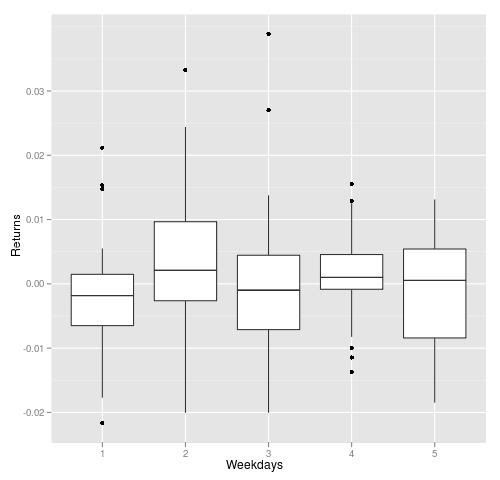Want to share your content on R-bloggers? click here if you have a blog, or here if you don't.

Inspired by CXO group report, I did a rerun of the same strategy on my data. Easter’s dates can be find at wikipedia. Overall, my results are similar to CXO group’s results.

In the graph below, I plotted daily returns on Easter week (Monday to Thursday) from 1982 to 2009. I prefer this way of showing things, where the range, minimum, maximum and mean of returns are presented.
It is clear, that only returns on Thursdays are above zero and t-test confirms that:
t = 2.235, df = 27, p-value = 0.03389. The rest is close to random.The graph below shows daily returns one week after Easter holidays. Although Monday looks like negative day, but it lacks significance:
t = -1.1517, df = 27, p-value = 0.2595 (should be less that 0.1).
The rest is noise.In summary, only returns on Thursdays have positive bias.

```#R-Language code to repeat this test.
require(quantmod)
require(xts)
getSymbols('^GSPC',from='1980-01-01')

GSPC.delta<-Delt(log(Cl(GSPC)))
GSPC.delta<-Delt(Cl(GSPC))

#returns during the week before Easter
#returns on Thursdays
nasa<-data.frame(as.double(GSPC.delta[(as.Date(easter,'%m/%d/%y')-3)]),4)
colnames(nasa)<-c('ret','weekday')

#Monday to Wednesday
for(i in 1:3)
{
tmp<-data.frame(as.double(GSPC.delta[(as.Date(easter,'%m/%d/%y')-(3+i))]),(4-i))
colnames(tmp)<-c('ret','weekday')
rbind(nasa,tmp)
nasa<-rbind(nasa,tmp)
}
t.test(nasa\$ret[nasa\$weekday==4])

require(ggplot2)
qplot(factor(as.numeric(nasa\$week)),as.double(nasa\$ret),data=nasa,geom = "boxplot",ylab='Returns',xlab='Weekdays')

#returns during the week after Easter

GSPC.delta<-Delt(Cl(GSPC))
nasa<-data.frame(as.double(GSPC.delta[(as.Date(easter,'%m/%d/%y')+1)]),1)
colnames(nasa)<-c('ret','weekday')
for(i in 2:5)
{
print(i)
tmp<-data.frame(as.double(GSPC.delta[(as.Date(easter,'%m/%d/%y')+i)]),i)
colnames(tmp)<-c('ret','weekday')
rbind(nasa,tmp)
nasa<-rbind(nasa,tmp)
}
t.test(nasa\$ret[nasa\$weekday==1]);t.test(nasa\$ret[nasa\$weekday==2])
qplot(factor(as.numeric(nasa\$week)),as.double(nasa\$ret),data=nasa,geom = "boxplot",ylab='Returns',xlab='Weekdays')```